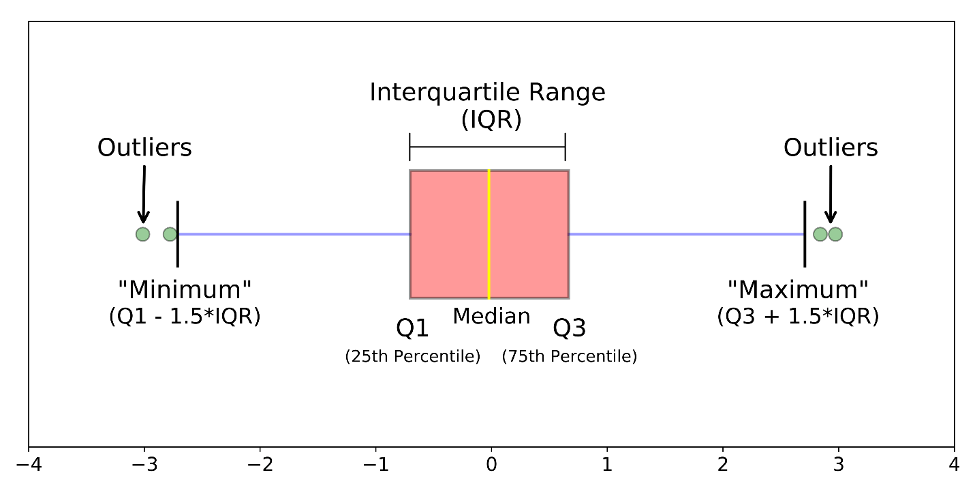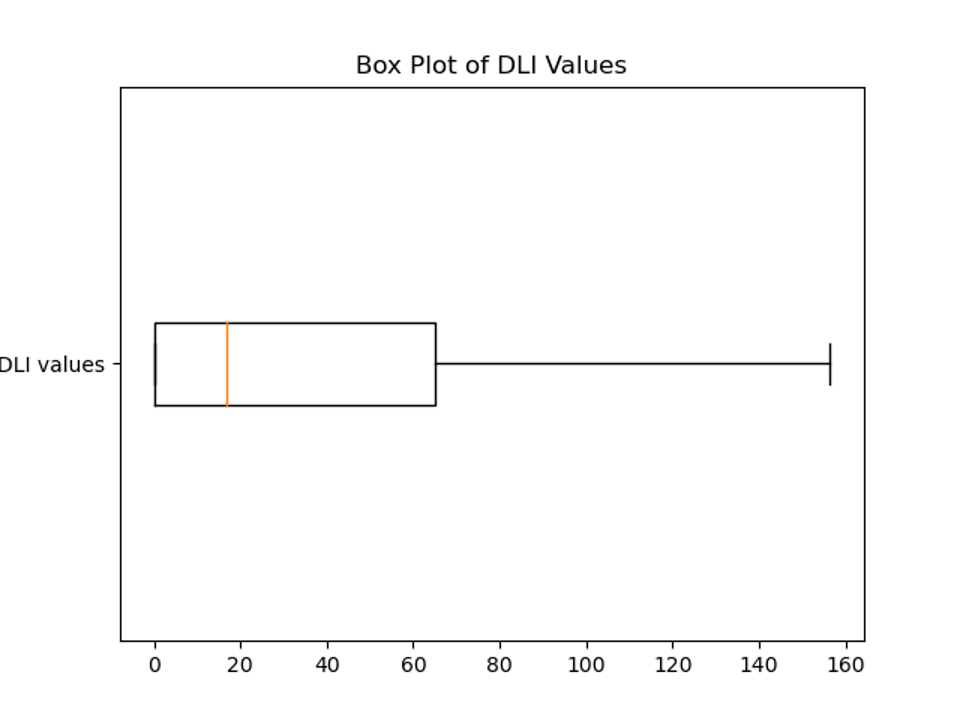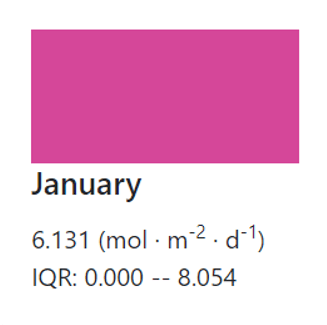## DLI Calculator

What is this?

This web app has been designed to help you determine daily light integral (DLI) values throughout the year for any location on Earth. It uses worldwide weather station data and algorithms to deliver the most accurate DLI values for your location.

What is DLI?

The Daily Light Integral (DLI) is a measure of how much daylight is delivered to a location over a 24-hour period. More specifically, it is defined as: “photosynthetic photon flux density (PPFD) integrated over a 24-hour period, typically coinciding with the 24 hours of a calendar day”. It is expressed as moles of light per square meter per day (mol * m-2 * d-1).

Why is it important?

Plant growth is driven by light. The ability to calculate DLI values for any location opens up many possibilities. Growers can estimate how much supplemental lighting will be needed throughout the year to allow a plant to reach its potential growth in a greenhouse, and they can also calculate the amount of shading needed for their crops. Outside the greenhouse, growers can determine whether crops grown in an open field will receive sufficient light during the course of a growing season to reach maturity.

How to use the app

You can use the app by either clicking on your location directly on the map or by entering geographical coordinates into the box above the map, and then clicking on submit.

1. Using the map

The map is simple to use. Move the map by clicking and dragging or touching the map if on mobile.

Zoom in with the + and – buttons or by using the scroll wheel, or by pinching if on mobile. There is a search function on the map, represented by the magnifying glass button. Clicking on it allows you to search for a location, and pressing enter or tapping the search term on mobile will bring you to that location. Note that searching for a location only brings you to that location, it does not refresh the DLI values for that location.

1. Generating a report

Once DLI values have been generated, an option appears to download a PDF report. This will output your results into a PDF file for your convenience. DLI values will be shown on the right, and your chosen location will be pinned to a map.

1. Toggle Stations

This button allows you to see all of the 2,000+ World Meteorological Organization (WMO) weather stations located around the world, represented by blue circles.

1. Geographic Coordinates

Note that latitude is positive in the northern hemisphere, and negative in the southern hemisphere. Longitude is positive east of the prime meridian, and negative west of the prime meridian.

Coordinates must be entered as latitude and longitude in decimal form. For example, Victoria, Canada would be entered as “48.42, -123.36”.

If you have coordinates available in degrees / minutes / seconds (DMS) form, you can convert them to decimal form using the formula:

Decimal degrees = degrees + (minutes/60) + (seconds/3600)

For example, if you have:

48° 25′ 42.3” N

123° 21′ 56.3” W

these become:

48 + (25/60) + (42.3/3600) = 48.42

-123 + (-21/60) + (-56.3/3600) = -123.36

You can also use an online coordinate converter, such as: https://www.pgc.umn.edu/apps/convert/.

Alternatively, simply zooming in as necessary and clicking on the map will show your latitude and longitude coordinates in the input box in decimal form.

CAUTION: If there are no weather stations located with 100 km of the chosen location, the predicted DLI values may not be accurate.

Greenhouses

The DLI values given by this app correspond to the amount of light received outdoors for a particular location. To estimate the DLI values inside your greenhouse, carry out the following:

1. Measure light intensity outdoors at noon on a clear day using a photometer.
2. Measure light intensity in your greenhouse at noon on a clear day using a photometer.
3. Calculate the percentage of light that is transmitted to your greenhouse by dividing your value obtained in Step 2 by the value obtained in step 1.
4. Multiply DLI values by that percentage calculated in step 3 to obtain your DLI values inside your greenhouse.

Example: Say we calculate 1500 lux outside and 750 lux inside a greenhouse. Then our value for step 3 is 750/1500= 0.5. Now we simply multiply every DLI value outputted for our location by 0.5 to get the DLI values for the inside of our greenhouse.

Show Interquartile Range (IQR)

On clicking the “show IQR” button, interquartile ranges for the displayed DLI values appear. The interquartile range is a metric that describes statistical dispersion. In other words, how much the data is spread out. The IQR is written in terms of percentiles. Percentiles are data points where a certain percent of the data is less than that data point. For example, the 20th percentile is the data point where 20 percent of the data is less than that data point. In an IQR, we have the 25th percentile and the 75thpercentile, labeled Q1 and Q3 respectively. The IQR is what data lies between the 75th percentile and the 25th percentile, in other words where 50 percent of the data lies in the dataset. This gives us an idea of how much variation there is in the data used to calculate DLI values with the DLI Calculator. The average DLI is given first, then the IQR tells us what other DLI values we can expect to see as well.

Example: The average DLI value for the month of January is calculated to be

6.131 (mol * m-2 * d-1). The interquartile range is 0 (mol * m-2 * d-1) to 8.054 (mol * m-2 * d-1) where 0 is Q1 and 8.054 is Q3 respectively. This information would be displayed as below. So, 50 percent of the DLI values in January lie between 0 and 8.054 (mol * m-2 * d-1). Note that this 50 percent interval can contain multiple 0 values.

To visualize the IQR, a box plot is used. This is commonly known as a box and whiskers plot. See the example box plot. A sample weather station’s DLI values for one month have been plotted on a box plot. We can see that the IQR is between 65 and 0, the “minimum” is 0, the median is around 17, and the “maximum” is around 156.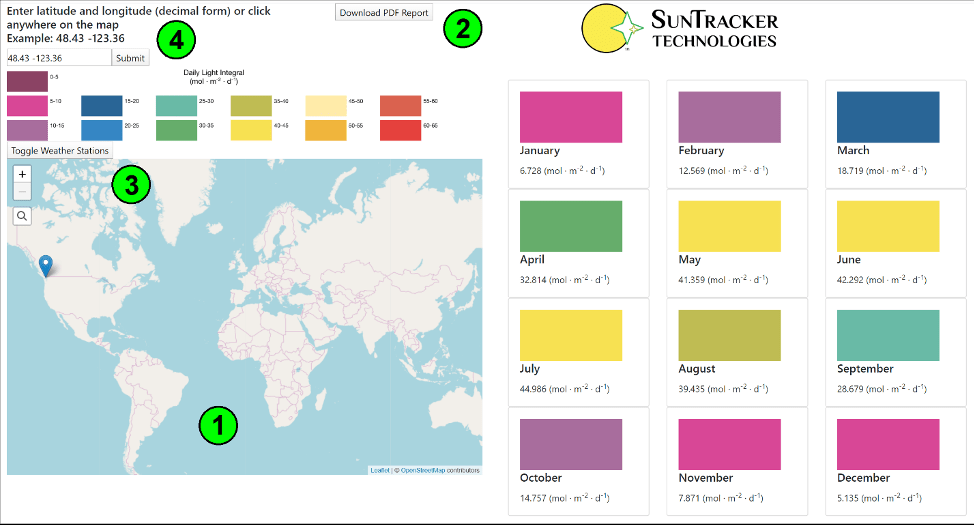Fig. 1 – DLI Calculator

 Faust, J. E., and J. Logan. 2018. “Daily Light Integral: A Research Review and High-resolution Maps of the United States,” HortScience 53(9):1250-1257.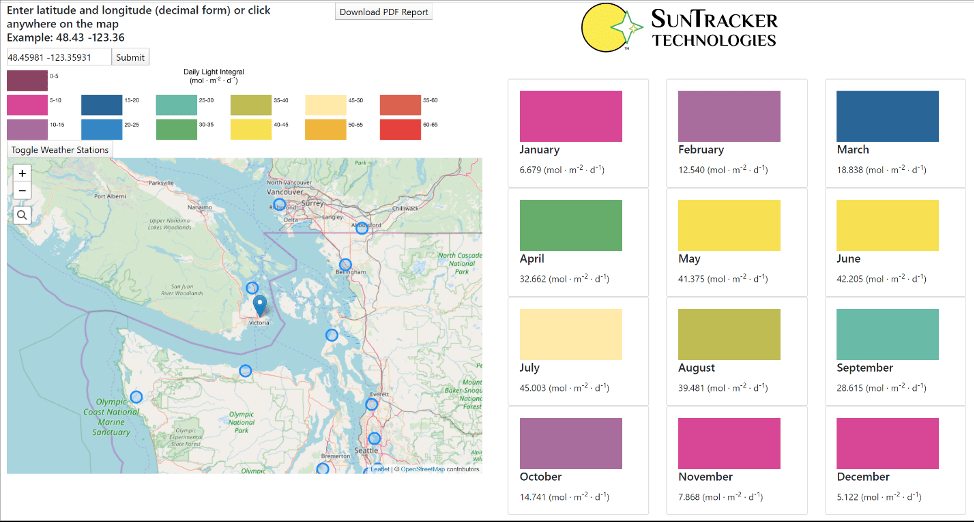Fig.2 – DLI Calculator

 It is important to remember that the amount of light incident upon the plant canopy in a greenhouse may be between roughly 40 and 60 percent of the amount of light incident on an open field adjacent to the greenhouse. The actual values depend on the glazing transmittance, the greenhouse roof design, the building orientation, whether the glazing is transparent or translucent, the amount of cloud cover, and more. SunTracker Technologies’ Cerise365 + Greenhouse Designer can calculate accurate DLI values inside greenhouses, hybrid vertical farms, and building atria.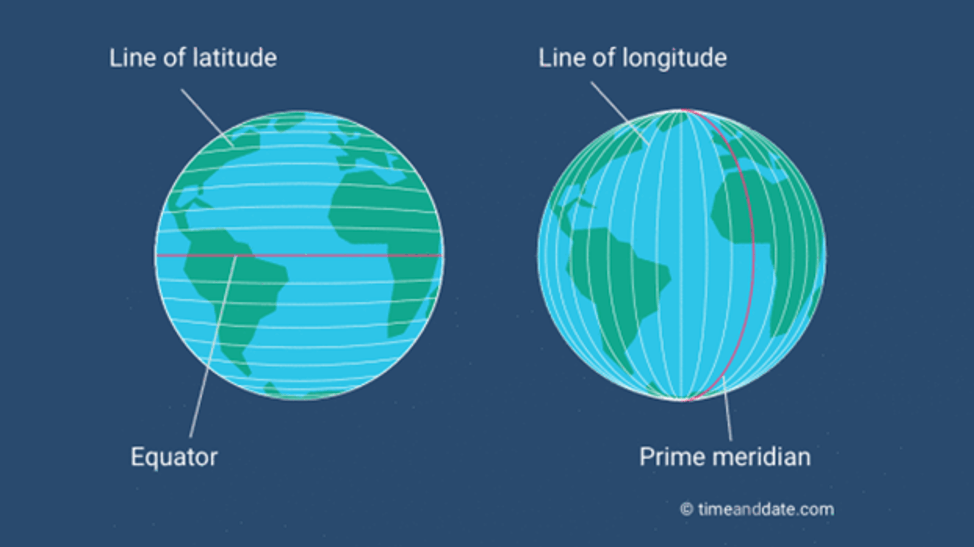Fig. 3 – Geographic coordinates orientation